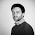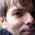Tuesday, 29 January 2013

False Discovery Rates and Large Files

Welcome to my first, of what will hopefully be many, blog post.  I'm currently a Computational Biologist at the University of Glasgow based within the Beatson Institute for Cancer Research.

While I don't officially represent either institution this blog will aim to explain some of the things that I do and to share information or code which might be useful to others working within the field.

To start with I'll present a small problem I encountered a few months ago but which I think demonstrates how powerful UNIX tools can be for tackling bioinformatics problems.

Task: I have a large file where I wish to calculate the FDR of a single column.

Problem: When the file is loaded into R in order to use the p.adjust() function the R process uses more memory than I want it to.  A file which is 6.5GB on disk; with a mixture of strings, integers and floats; peaks at about 15GB to read in using R's read table function.

I've got a pretty beefy machine for doing bioinformatic analysis on but memory is still a finite resource and I'm often working on more than one project at a time.  As such I quite often want to control the amount of memory that I use for various pipelines.

Solution: Reimplement the FDR algorithm using GNU tools.

#!/bin/sh

if [ $# -ne 2 ] then echo "usage: PtoFDR file column" exit 1 fi export LC_ALL=C sort -S 10G -k"$2","$2"gr$1 | \
awk -v col=$2 -v numrows=wc -l$1 |  awk '{print $1}' ' function min(a,b) { if (a <= b) return a else return b } BEGIN { cummin = 1.0; OFS="\t"; } { cummin = min(cummin,$col*(numrows/(numrows - NR + 1)));
$col = cummin; print }'  This is just an reimplementation of the Benjamini-Hochberg procedure found in p.adjust(). This is a step-up procedure so starts from small test statistics (large p-values) and iterates through them in increasing test statistic order (decreasing p-values) -- hence the reverse sort. You can control the amount of memory available by adjusting the -S parameter given to sort (set to 10G above). This will limit the main memory buffer size (thus forcing sort to use temporary files on disk to do the sort). The awk command processes the sorted file line by line so should use a minimal amount of memory. As it's just a simple shell script it should neatly slot into many bioinformatics pipelines. Notes: • This will not preserve the original sort order of your input file. If you want to do this then add a column with an incrementing counter 1..n to your file which you can then use to resort afterwards. • The column number parameter is 1-based (i.e. 1,2,3 rather than 0,1,2). • The P-value is replaced with the FDR(p) in the same column. This prevents the file format from changing but if you need to keep the original p-value as well then you will need to change the script to add a new column. • LC_ALL is set to C in the script above which has tremendous performance advantages during the sort but which limits your input files to, essentially, ANSI if you want to avoid codepoint problems. • One alternative approach if you want to stay within an R environment is to use the mmap package to avoid loading the file into memory. Update: Tommaso Leonardi contributed the following code which adds the FDR as an additional column instead of replacing the original p-value as my approach did. #!/bin/sh if [$# -ne 2 ]
then
echo "usage: PtoFDR file column"
exit 1
fi

export LC_ALL=C
sort -S 10G -k"$2","$2"gr $1 | \ awk -v col=$2 -v numrows=wc -l $1 | awk '{print$1}' '
function min(a,b)
{
if (a <= b)
return a
else
return b
}

BEGIN { cummin = 1.0; OFS="\t"; }
{
cummin = min(cummin,$col*(numrows/(numrows - NR + 1))); for (i = 1; i <= col ; i++){ printf$i"\t"
}
printf cummin"\t";

for (i = col+1; i <= NF; i++){
printf $i"\t" } printf "\n" }'  Update 2: I've quickly made the following script which can use either of the two PtoFDR approaches above but will then resort your file back to its original order. It works by adding the row number to the beginning of every line, running an existing PtoFDR script then resorting the file by row number and removing that column. It uses a few more temporary files than is strictly necessary but this guarantees that memory usage won't ever exceed what you set for -S (otherwise if you pipe two sorts together then you can use double the memory). #!/bin/sh if [$# -ne 2 ]
then
echo "usage: PtoFDR.sorted file column"
exit 1
fi

export LC_ALL=C
awk 'BEGIN {OFS="\t"; }{print FNR,$0}'$1 > $1.tmp PtoFDR$1.tmp calc $2+1 >$1.tmp2
sort -S 10G -k1,1n $1.tmp2 | cut -f2- rm$1.tmp \$1.tmp2


1.Great post and very useful code, thank you!
I've modified you script so that it adds the FDR column next to the p-value one. I tried (twice) to add the code to the comment but Blogger is messing with the code. If you want I can send it via email ;)

cheers!

2.